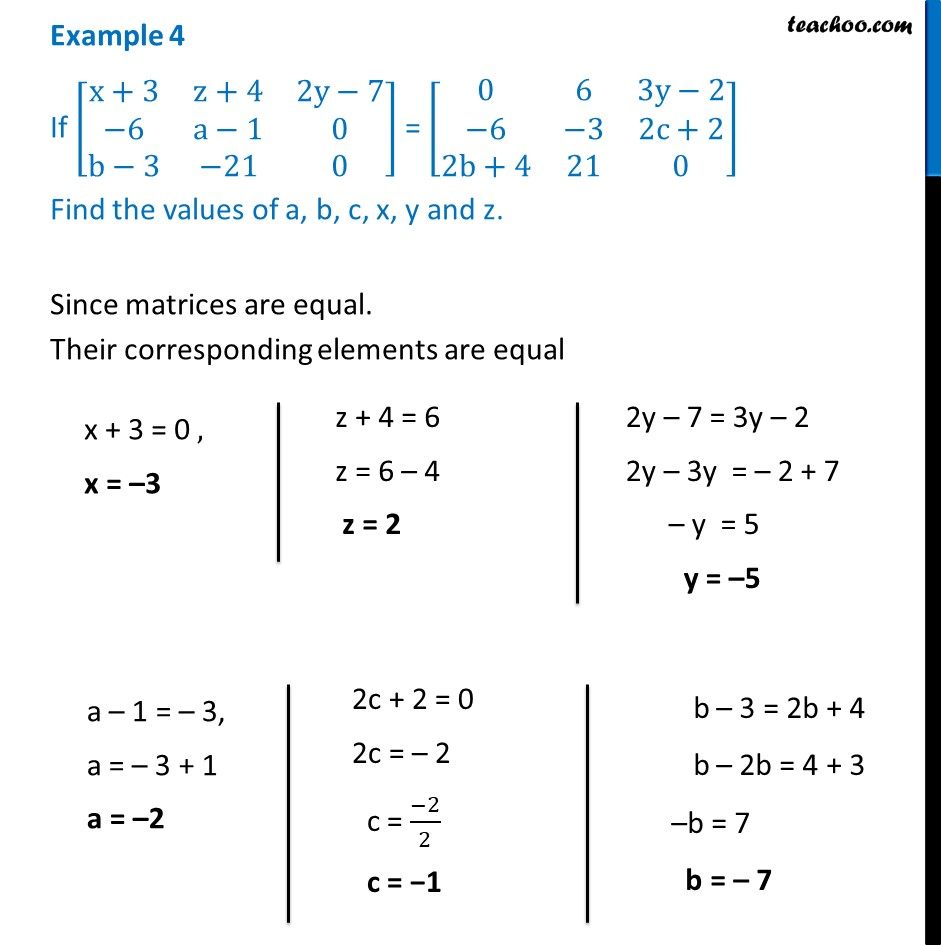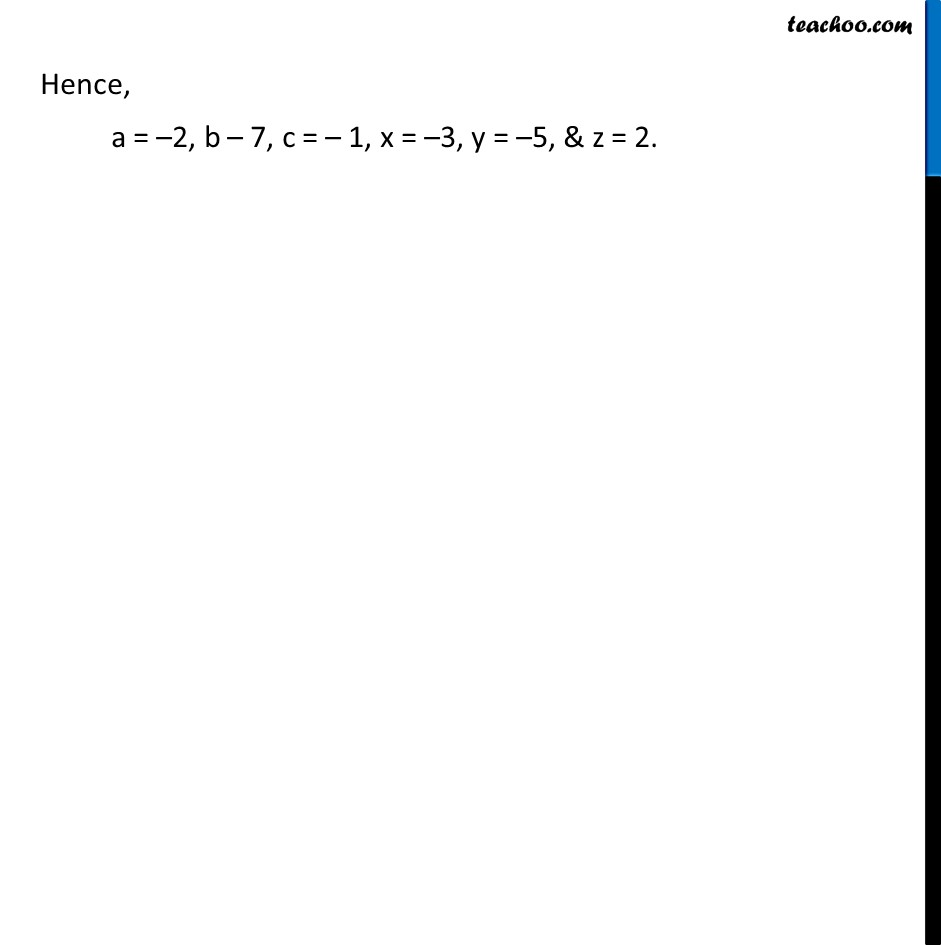Examples

Chapter 3 Class 12 Matrices
Serial order wiseLearn in your speed, with individual attention - Teachoo Maths 1-on-1 Class

### Transcript

Example 4 If [■8(x+3&z+4&2y−7@−6&a−1&0@b−3&−21&0)] = [■8(0&6&3y−2@−6&−3&2c+2@2b+4&21&0)] Find the values of a, b, c, x, y and z. Since matrices are equal. Their corresponding elements are equal x + 3 = 0 , x = –3 z + 4 = 6 z = 6 – 4 z = 2 2y – 7 = 3y – 2 2y – 3y = – 2 + 7 – y = 5 y = –5 a – 1 = – 3, a = – 3 + 1 a = –2 2c + 2 = 0 2c = – 2 c = (−2)/2 c = −1 b – 3 = 2b + 4 b – 2b = 4 + 3 –b = 7 b = – 7 Hence, a = –2, b – 7, c = – 1, x = –3, y = –5, & z = 2.